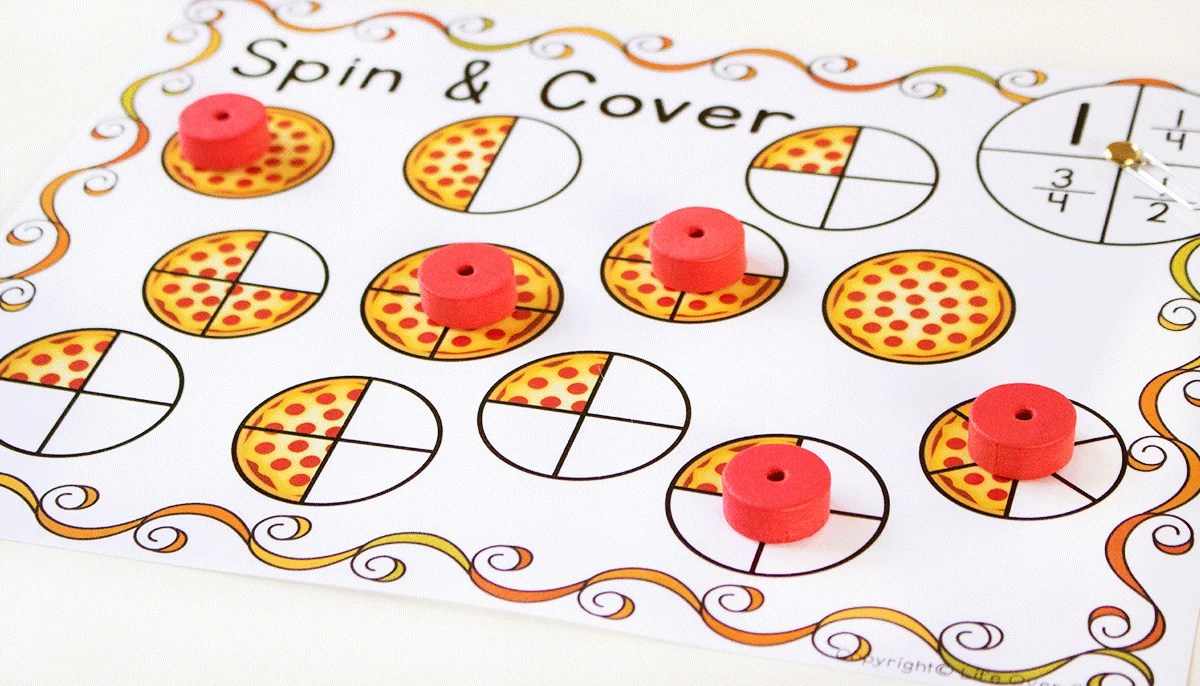# Happy Math Month-Math activities

Home > Blog  > Happy Math Month-Math activities

### Happy Math Month-Math activities

The month of April is the international math month. Educators all over the world encourage their students to learn math by introducing fun activities in their classrooms.

Below are a few math activities that we love here at Budding Genius Academy

Hopscotch MathUsing a piece of chalk, draw a hopscotch grid on the pavement mimicking a calculator layout. Ask the students to form a line and one by one, give them a simple operation (e.g. 5+3, 7–3). Students should take turns hopping on each element of the equation in the correct order, landing finally on the answer.

call out a number and ask the students to hop on any equation that equals to that number. For a fun twist, ask students to hop on one leg for odd numbers, and two legs for even numbers.

## Pizza fractions Spin and cover

This activity help the students to visualize the key concepts.

Create an instruction sheet with 8 different fractions on each (you can create several so different students get a different set). Students should create a pizza (using construction paper, or even the inside of an empty pizza box) and decorate the toppings to represent each fraction or can use spin and cover cards.

For example, if they had a third as a fraction,  they will spin and cover the matching fraction